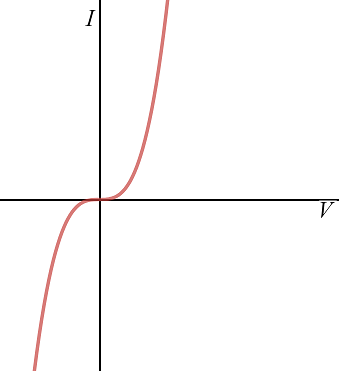Courses
Courses for Kids
Free study material
Free LIVE classes
More# The I-V characteristic shown in figure representsA) Ohmic conductorsB) Non-Ohmic conductorsC) InsulatorsD) SuperconductorsVerified
289.2k+ views
Hint: The above problem can be solved by using the concept of the conductors, Insulators and superconductors. The graphical representation of the voltage and current is called the I-V characteristics. The conductors, Insulators and superconductors behave in different manner for variation in the current with the variation in the voltage.

The conductors allow the flow of electrons in the wire due to applied voltage at room temperature. The conductors can be categorized into two types. First is the Ohmic conductor and other is the non-Ohmic conductor.
The current in the Ohmic conductor varies proportional to the applied voltage. The I-V characteristics of the Ohmic conductors are the straight line, so the option (A) is incorrect.
The current in the Ohmic conductor does vary proportional to the applied voltage. The I-V characteristic of the Ohmic conductors is not the straight line, so the option (B) is correct.
The insulator does not allow the flow of the electrons due to applied voltage and current does not conduct in the insulators at room temperature. The I-V characteristic of the insulators is the straight line that lies on the voltage axis, so the option (C) is incorrect.
The superconductors show high variation in the current at very low voltage. The I-V characteristics for the superconductors are the vertical line parallel to the current axis of the I-V graph, so the option (D) is incorrect.

Additional Information: The insulator requires very high voltage for the flow of current because the electrons in the material of the insulator remain in an isolated state at room temperature. The superconductor requires very small voltage for the flow of current because the electrons in the material of the superconductor remain in the excited state at room temperature.

Note: Draw the graph between the current and voltage to find the I-V characteristics. The I-V characteristics describe how the current behaves for the variation in the voltage.
Last updated date: 29th May 2023
Total views: 289.2k
Views today: 6.46k